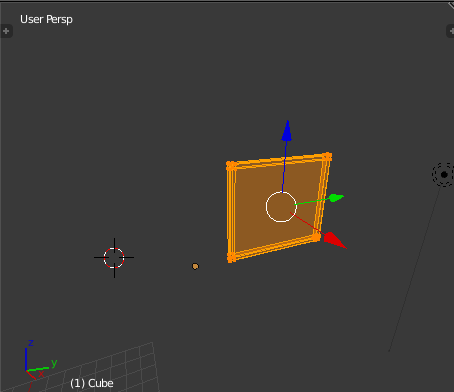# how do i deselect vertices

• im trying to select the vertices on left side of my object, move them, and then select the right side, plz help, this is what i have:

import bpy
import bmesh

middley = 13.65
middlez = 13.9

def select_left_side():
bpy.ops.object.mode_set(mode=“OBJECT”)
for item in bpy.data.objects:
if item.type == ‘MESH’:
for vertex in item.data.vertices:
if vertex.co.y < middley:
vertex.select = True
bpy.ops.object.mode_set(mode=“EDIT”)

def select_right_side():
bpy.ops.object.mode_set(mode=“OBJECT”)
for item in bpy.data.objects:
if item.type == ‘MESH’:
for vertex in item.data.vertices:
if vertex.co.y > middley:
vertex.select = True
bpy.ops.object.mode_set(mode=“EDIT”)

select_left_side()
bpy.ops.transform.translate(value=(0, 1.5, 0))
bpy.ops.mesh.select_all(action=‘DESELECT’)
select_right_side()

this is what happens after running this script:i want to deselect the left side, select the right side and continue moving the vertices

help me plez :((